### Choose language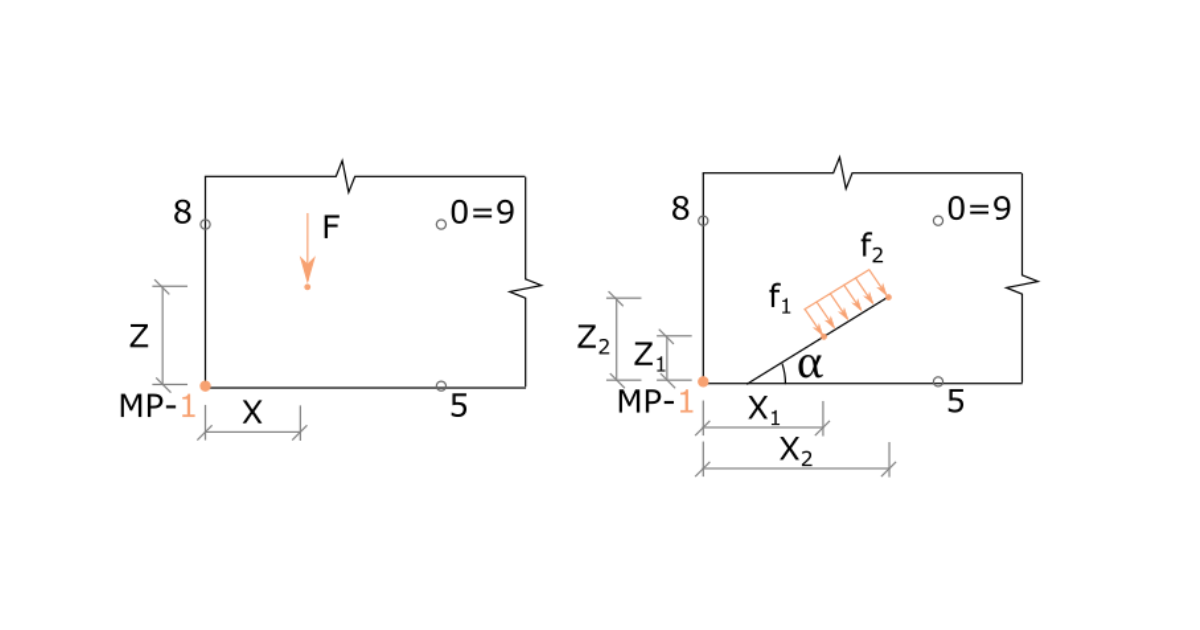# General description of Load impulses in Detail application

In this article, you will learn about load impulses that can be set in the IDEA StatiCa Detail application. We will explain what are the options, and describe them individually. So, in the end, you shall be sure what is the difference between them and when and how to use them for your design.
$$Four types of load impulses can be defined for reinforced concrete discontinuity regions: Point load Line load Surface load Self-weight Now, let's have a look at each of the options.Point loadThis simple load impulse is defined by its value, direction, inclination, position, and effective radius. The way how a point load is set can influence the method of how the load is applied to the model.The first option is to set the load on edge. See the figure below.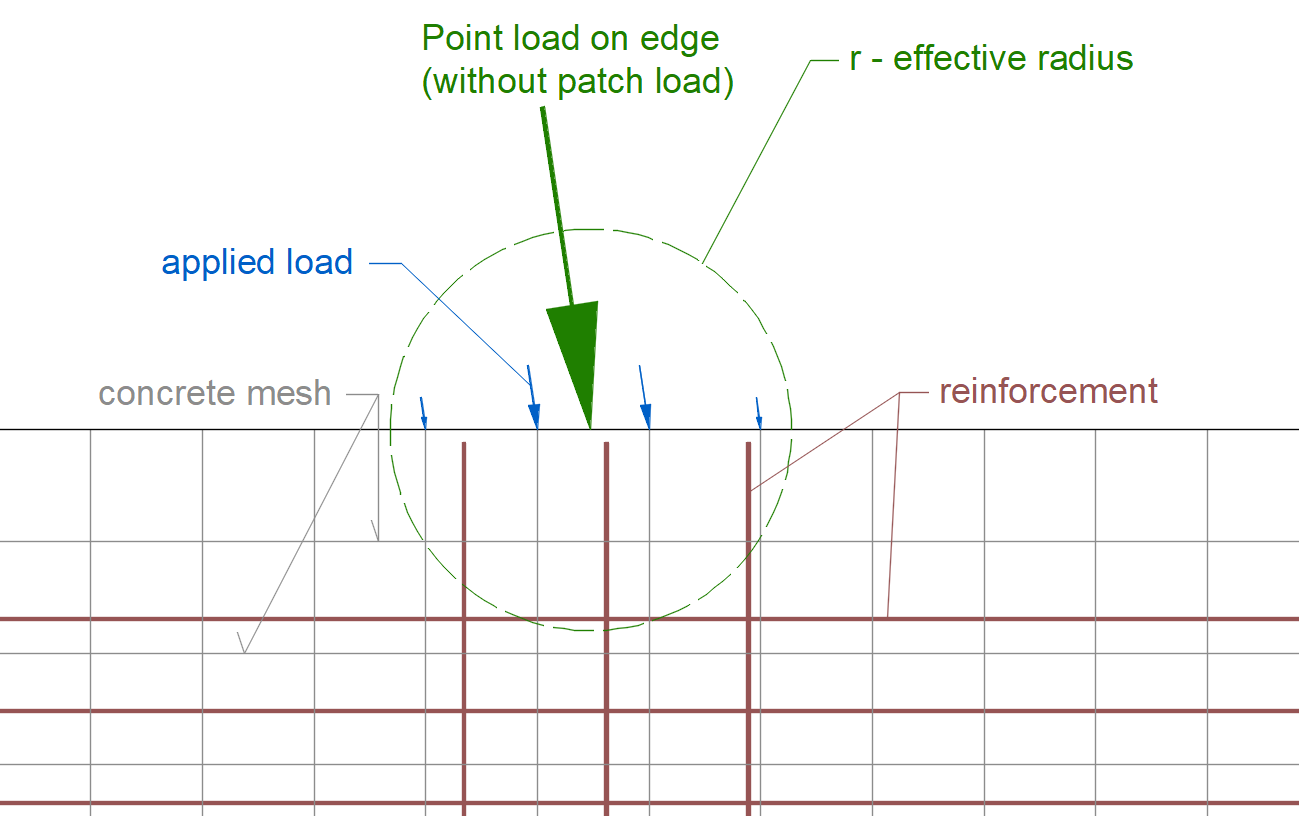The second option is to set the load generally into the model. The value of every applied load is calculated by weighted average where the weight is the distance from a point of application. In other words, the applied loads are variably distributed, not uniformly.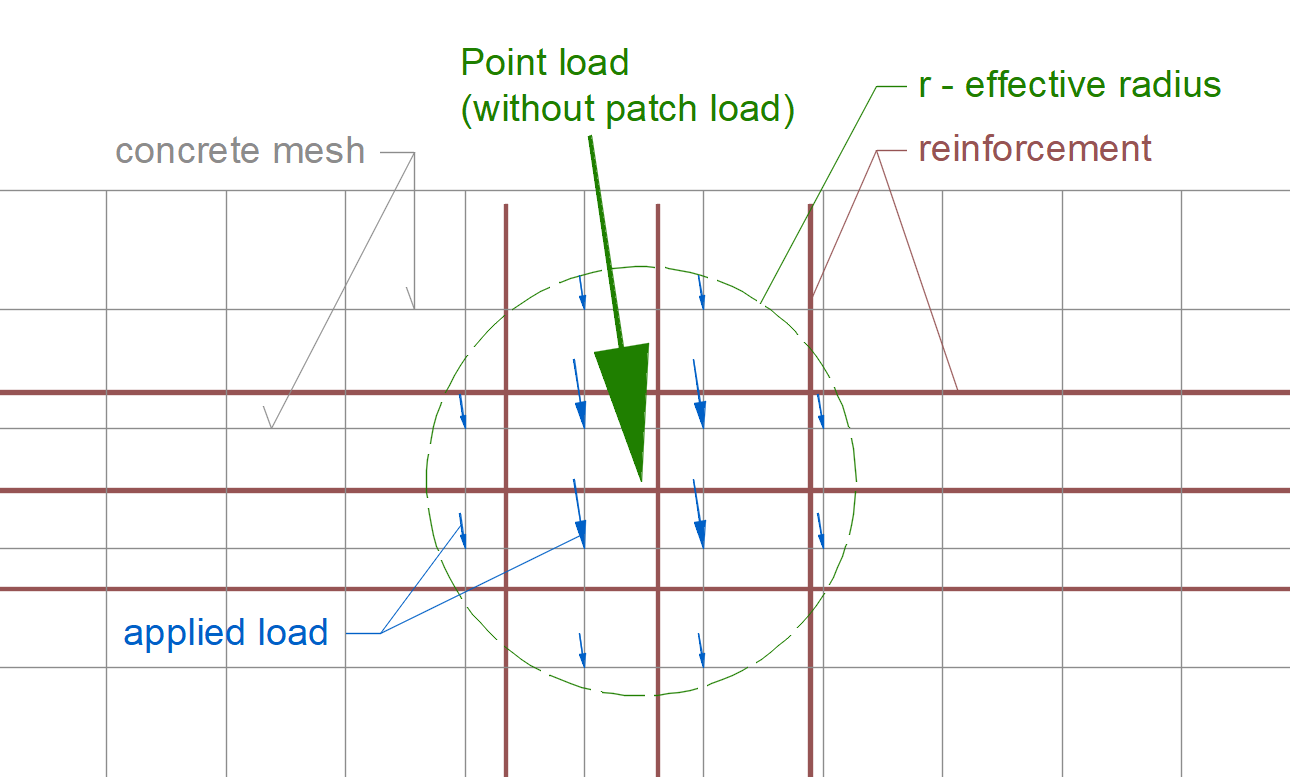The third option is to adjust the load to the patch load - one of the load-transferring devices, by using the functionality in the Geometry section.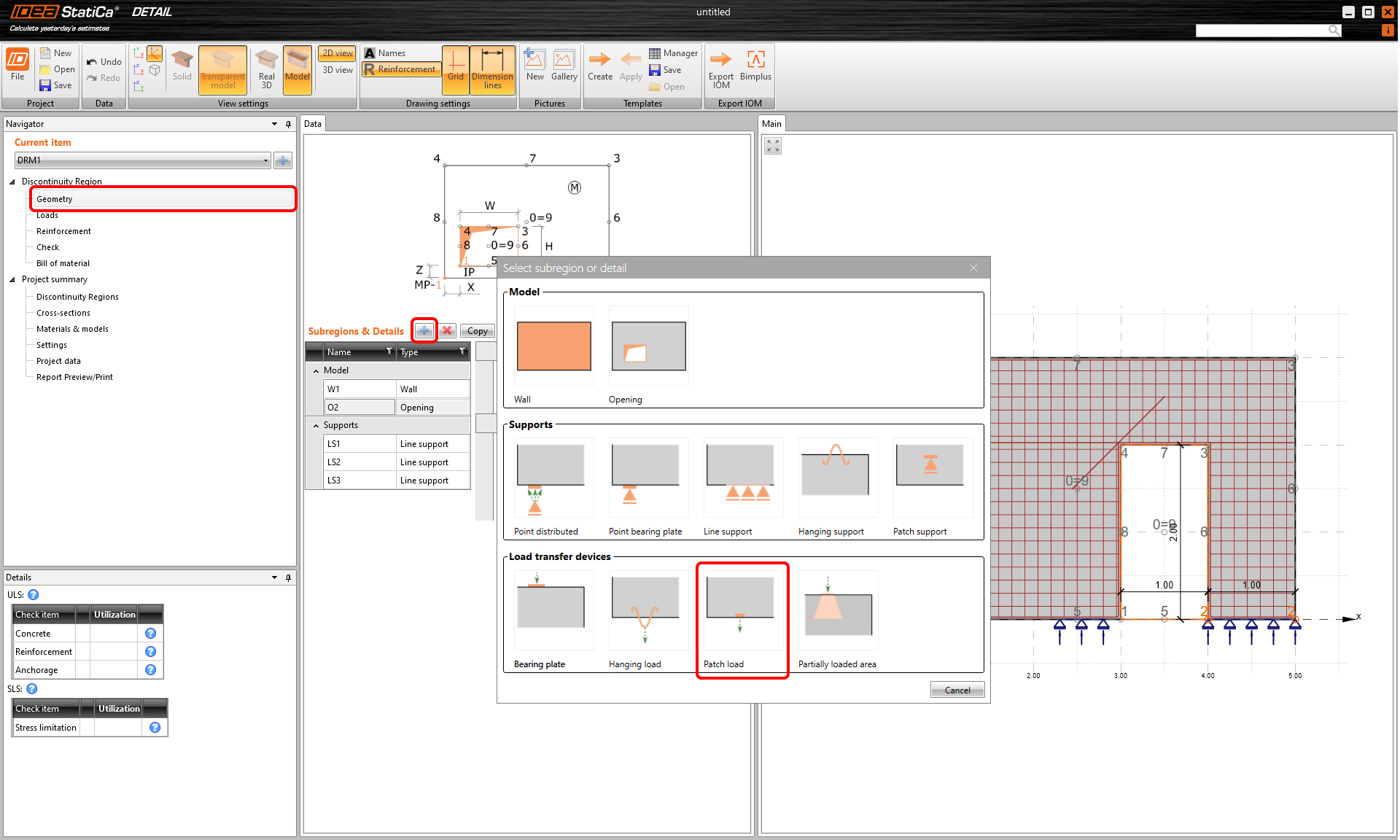The load application is shown in the image below.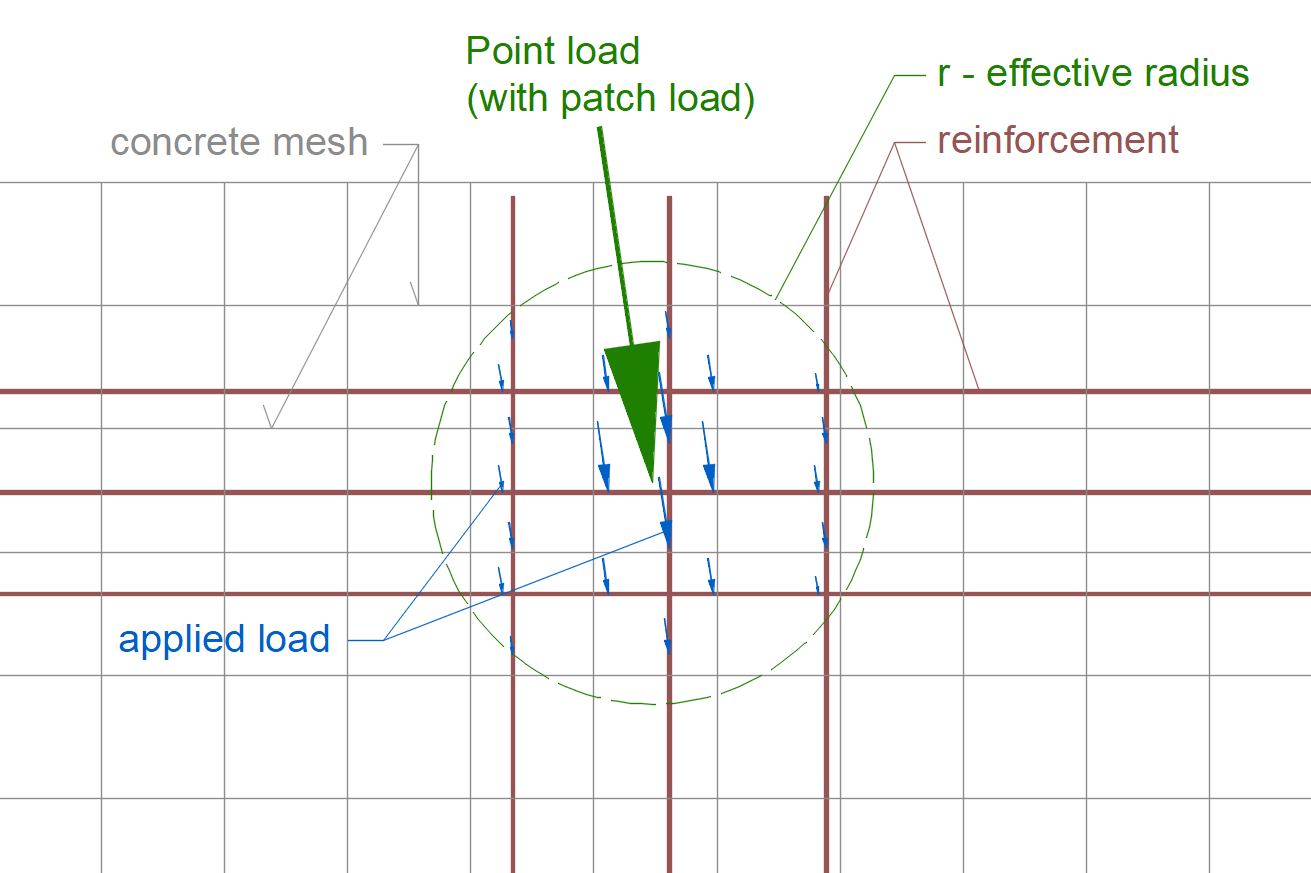Let's compare the images for the load placed into the model, and set using the patch load. Without patch load, the point load is applied to the elements of the concrete mesh. On the other hand, with patch load, the point load is applied straight to the reinforcement bars.The patch load can be connected to the adjacent reinforcement automatically by the software. However, for that, you have to turn on the checkbox for the corresponding bars in the Reinforcement section.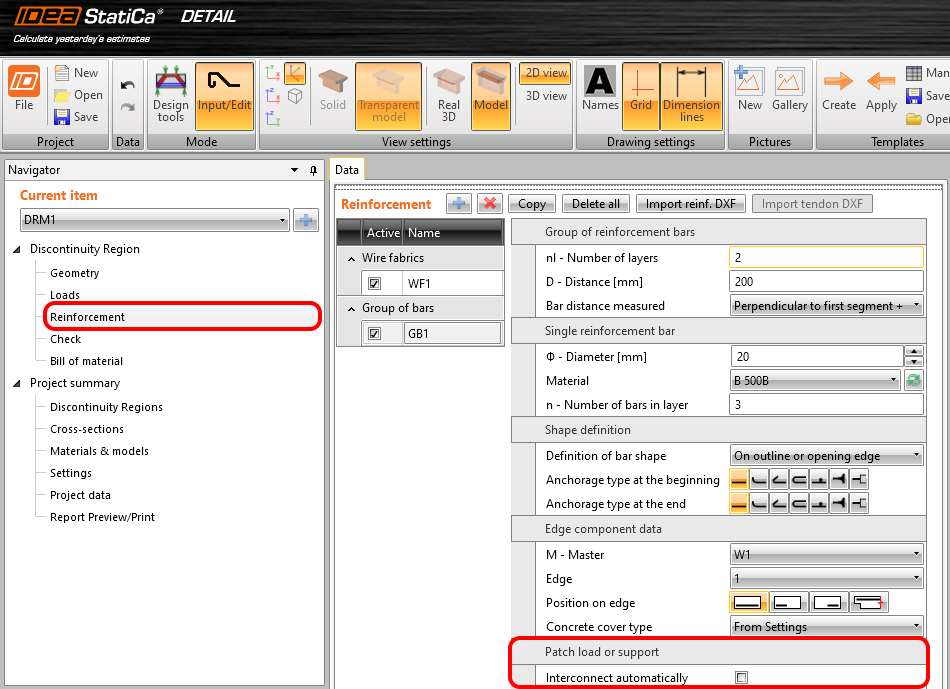In the figure below, you can see the case where the horizontal reinforcement was excluded from the patch load area, hence not considered for the load transmission.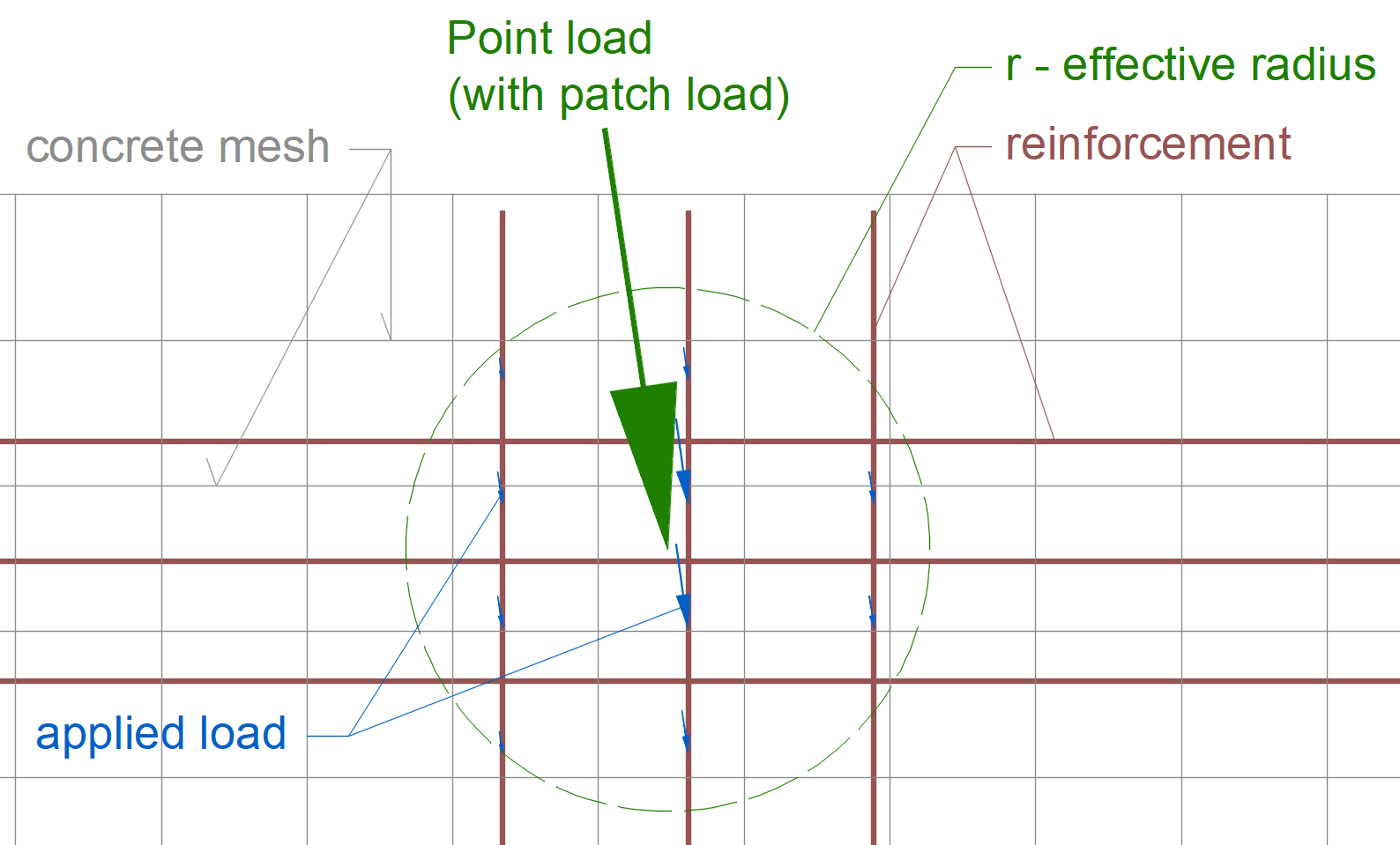The point loads can also be transferred via some other load-transmitting devices like bearing plates, hanging loads, and more. Do you want to know more about that? Read the article Support and load transmitting components.The main principle is that point loads are applied to the model by elastic links with different stiffnesses. The stiffnesses are evaluated by weighted averages based on the length of the links. These links are connected to the points of the concrete or reinforcement mesh (where the blue arrows were drawn) and transfer the loads to the structure.Line loadLine load can be defined as uniform or trapezoidal. The used type depends on the numerical load definition. When applying the linear line load, simply enter only one value to the corresponding cell in the data window. Nevertheless, if you need to specify the trapezoidal load, fill in values at one and the other end of the load. See the practical examples: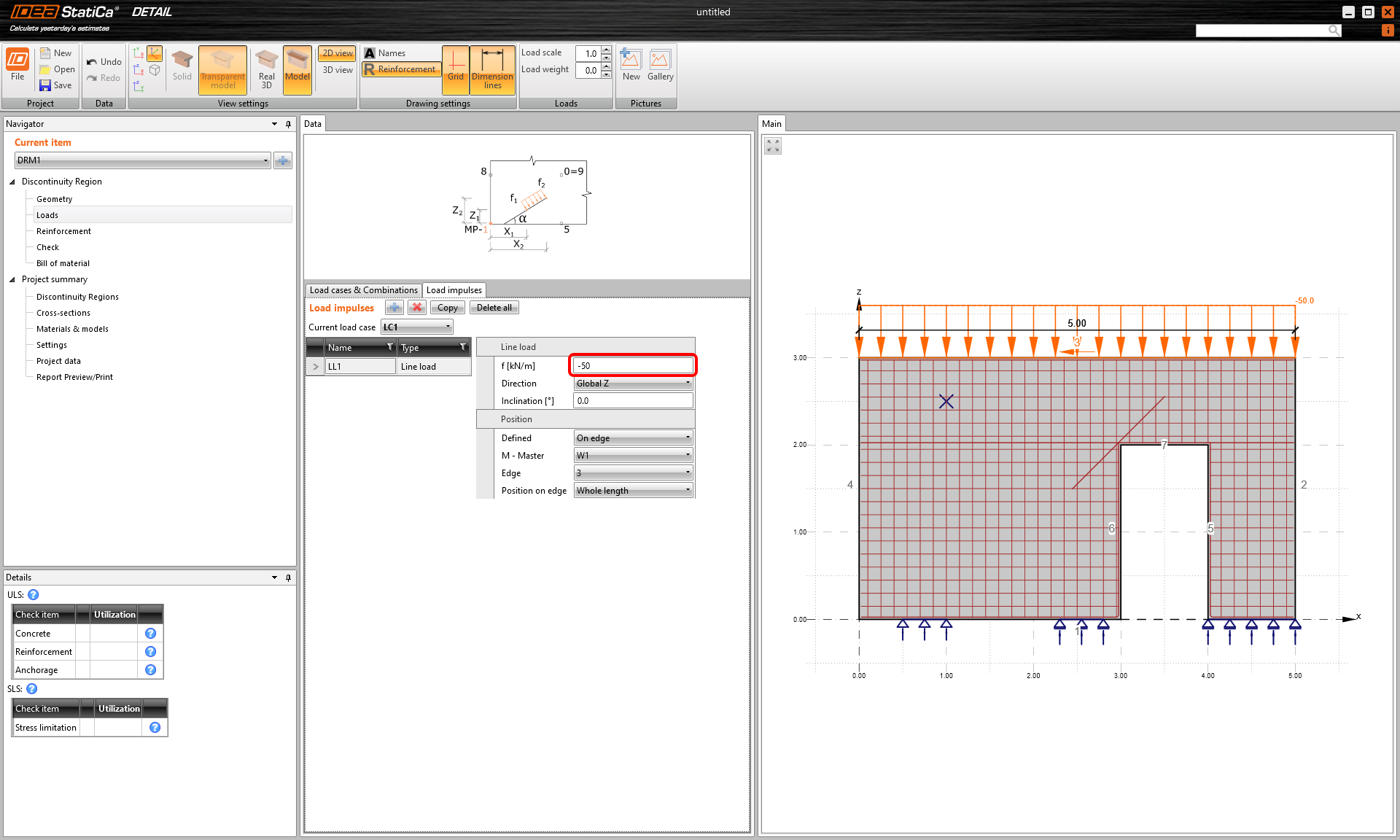Example of a uniform load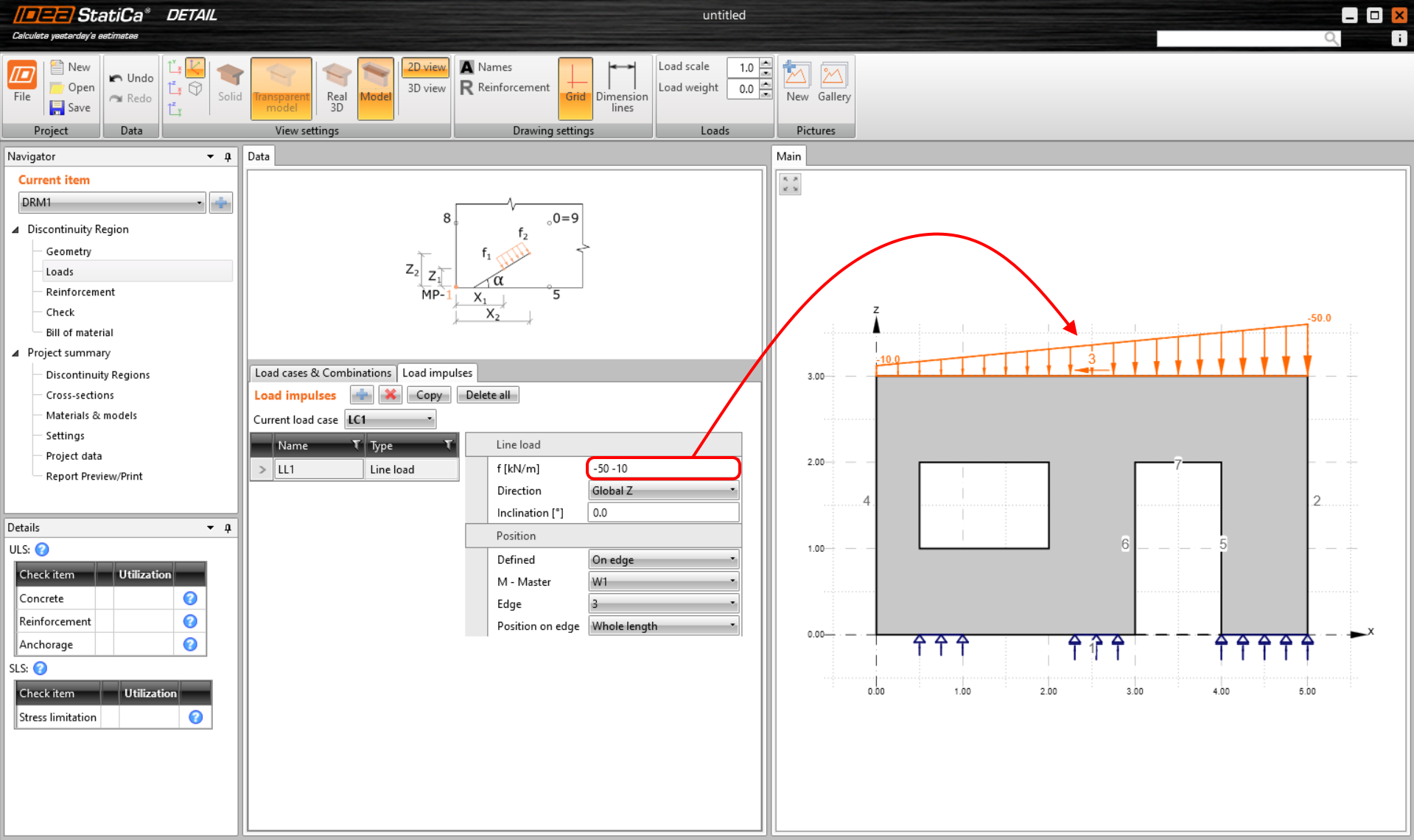Example of a trapezoidal loadThe principle of applying the load is almost identical to the point load.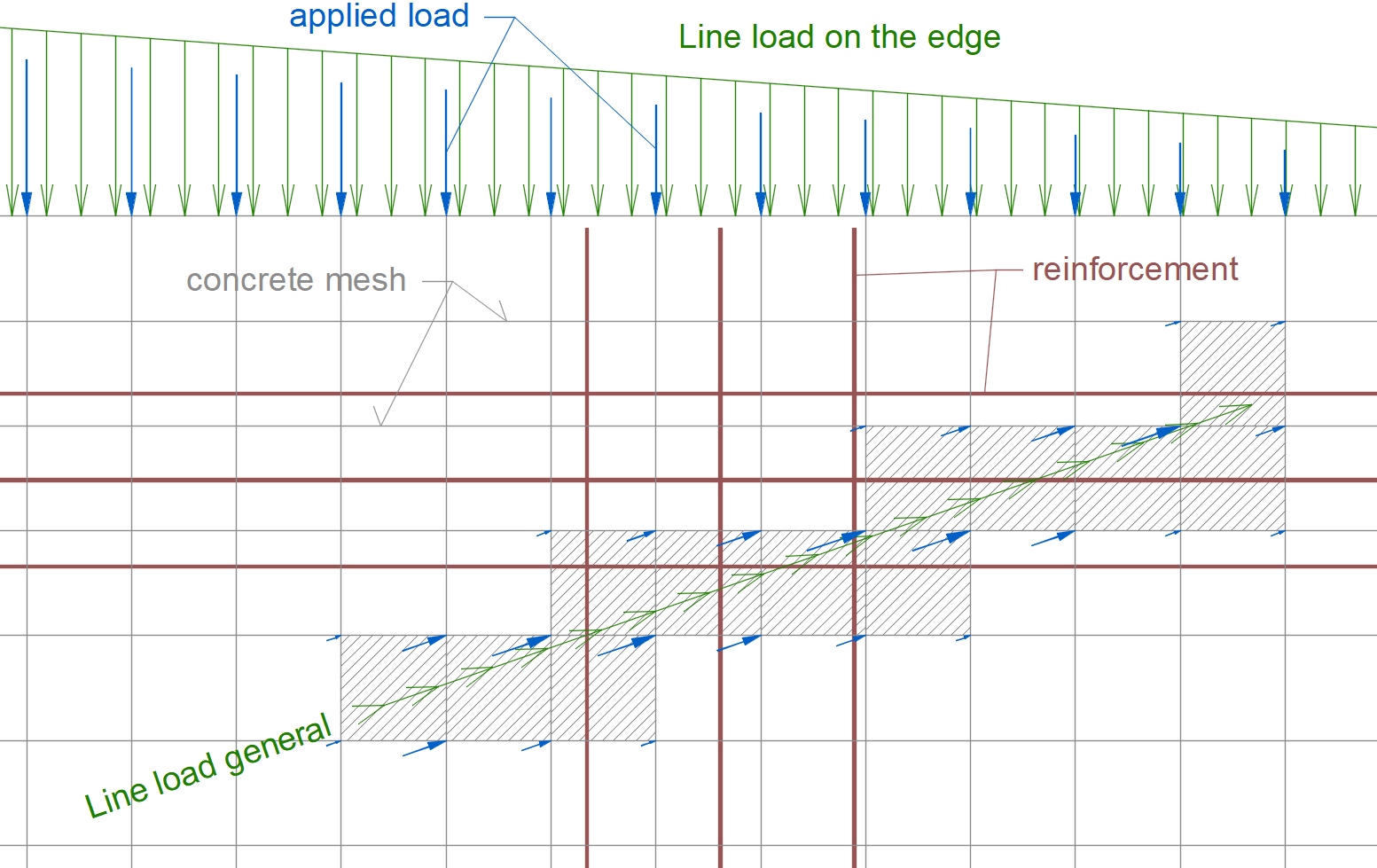Surface loadThe application of the surface load works the same way. It is applied to the nodes of the elements of the concrete structure's mesh.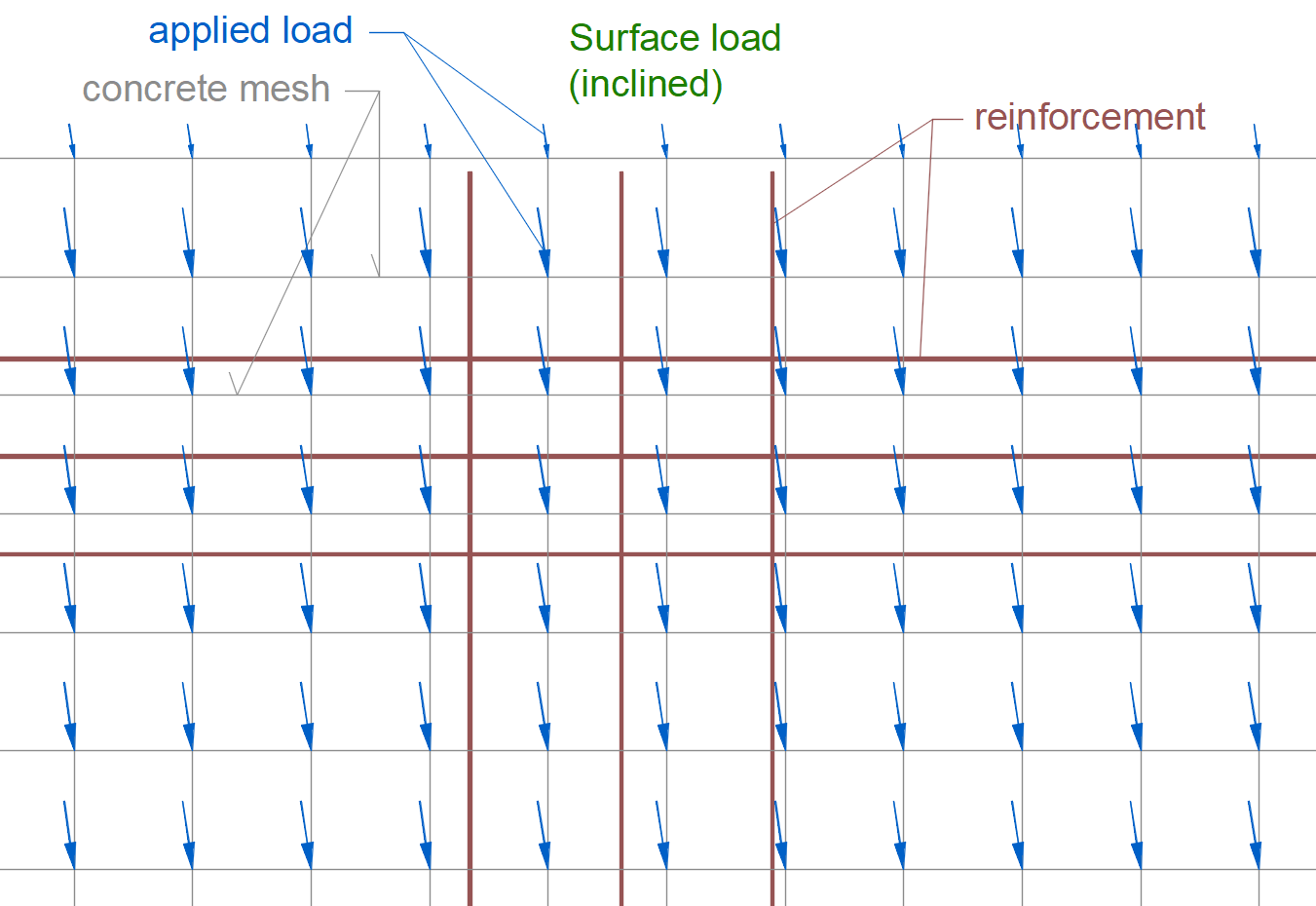Self-weightThe self-weight is a version of the surface load. The intensities are automatically calculated according to the selected cross-section.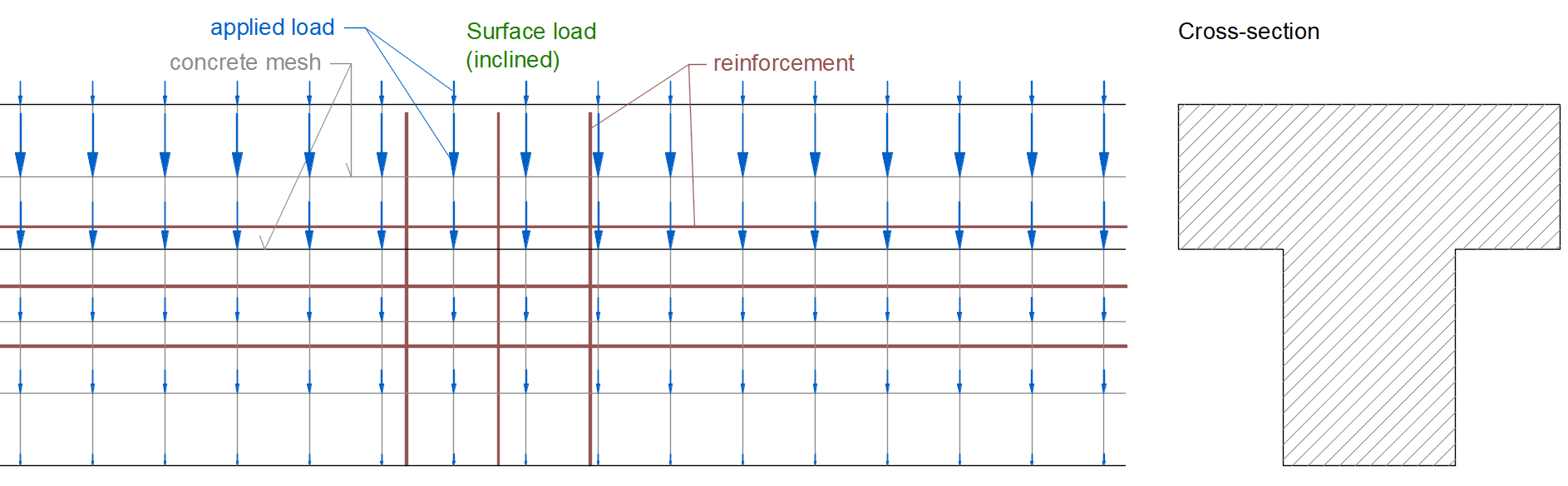$$